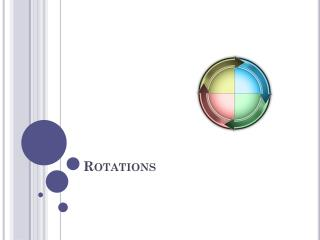# Rotations - PowerPoint PPT PresentationDownload PresentationRotations

RotationsDownload Presentation## Rotations

- - - - - - - - - - - - - - - - - - - - - - - - - - - E N D - - - - - - - - - - - - - - - - - - - - - - - - - - -
##### Presentation Transcript

1. Rotations

2. A rotation is a transformation in which a figure is turned about a fixed point. The fixed point is called the center of rotation. Rays drawn from the center of rotation to a point and its image form an angle called the angle of rotation. The angle of rotation can be measured clockwise (CW) or counterclockwise (CCW).

3. Can you describe the rotation? Is this the only way to describe the rotation?

4. A rotation about a point P through x° is a transformation that maps every point Q in the plane to a point Q’, so that the following properties are true. If Q is not point P, the PQ = Q’P and m<QPQ’ = x°

5. If Q is the point P, then Q = Q’.

6. A rotation is an isometry!

7. practice #1

8. Rotations about the origin 90° counterclockwise about the origin

9. 180° counterclockwise about the origin

10. 270° counterclockwise about the origin

11. Practice #2 Rotate ∆ABC with vertices A(2,-1) , B(4,1), and C(3,3) by 90° about the origin.

12. Practice #2 Rotate ∆ABC with vertices A(2,-1) , B(4,1), and C(3,3) by 180° about the origin.

13. Practice #3 The London Eye observation wheel has a radius of 67.5m and takes 30 minutes to make a complete rotation. A car starts at position ( 34, 59 ). What are the coordinates of the car’s location after 15 minutes? Justify your answer. Find the coordinates of the location of the observation car after 7.5 minutes.

14. A figure in the plane has rotational symmetry if the figure can be mapped onto itself by a rotation of 180° or less.

15. Let’s extend this topic… If lines k and m intersect at point C, then a reflection in k followed by a reflection in m is a rotation about point P. The angle of rotation is 2x°, where xis the measure of the acute or right angle formed by k and m.

16. Solve for each variable.

17. Classwork and Homework Handout 7.3A and 7.3B